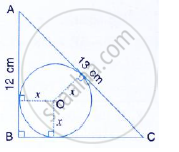Share

# Abc is a Right Angles Triangle with Ab = 12 Cm and Ac = 13 Cm. a Circle, with Centre O, Has Been Inscribed Inside the Triangle. Calculate the Value of X, the Radius of the Inscribed Circle - Mathematics

Course

#### Question

ABC is a right angles triangle with AB = 12 cm and AC = 13 cm. A circle, with centre O, has been inscribed inside the triangle.
Calculate the value of x, the radius of the inscribed circle#### Solution

In ΔABC , ∠B = 90°

OL ⊥ AB, OM ⊥ BC and ON ⊥ AC

LBNO is a square

LB = BN =OL =OM =ON =X

∴ AL =12 -x

∴ AL = AN =12 -x

since ABC is a right triangle

AC^2 = AB^2 +BC^2

⇒ 13^2 = 12^2 + BC^2

⇒ 169 = 144 + BC^2

⇒ BC^2 = 25

⇒ BC = 5

∴  MC = 5-X

but CM=CN

∴  CN = 5-X

Now , AC = AN + NC

13=(12-x) + (5-x)\

13= 17 -2x

2x = 4

x=2 cm

Is there an error in this question or solution?